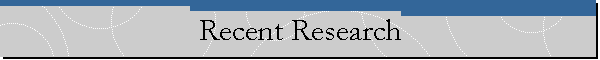##An integral domain D is called an h-local domain if every non-zero non-unit of D belongs to at most a finite number of maximal ideals of D and every non-zero prime ideal of D is contained in a unique maximal ideal. Call an ideal A unidirectional, pointing to a maximal ideal M, if M is the only maximal ideal containing A. I observed that D is h-local, if and only if, every proper principal ideal of D is expressible (uniquely) as a product of (mutually comaximal) unidirectional ideals of D. This observation, led to the study, using the star operations, of more general integral domains that have a factorization pattern similar to that of the h-local domains. (The paper based on this work has appeared in Houston J. Math.)

A foreign student, asked me to suggest a problem for his doctoral dissertation. I suggested that he should study integral domains all of whose overrings satisfy the ascending chain condition on principal ideals. He, and another Mathematician at the University of Bucharest, worked on this problem often asking me for guidance and help. It turned out that an integral domain has this property if and only if its integral closure is a Dedekind domain. A joint paper based on this work has been accepted for publication in , Communication in Algebra. The paper is expected to appear in the Fall of 2000.

back